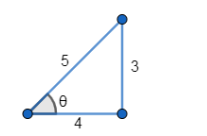Deepak Scored 45->99%ile with Bounce Back Crack Course. You can do it too!

# Prove that:

Question:

Prove that:

$\cos ^{-1} \frac{4}{5}+\sin ^{-1} \frac{3}{5}=\sin ^{-1} \frac{27}{11}$

Solution:

To Prove: $\cos ^{-1} \frac{4}{5}+\sin ^{-1} \frac{3}{5}=\sin ^{-1} \frac{27}{11}$

Formula Used: $\sin ^{-1} x+\sin ^{-1} y=\sin ^{-1}\left(x \times \sqrt{1-y^{2}}+y \times \sqrt{1-x^{2}}\right)$

Proof:

$\mathrm{LHS}=\cos ^{-1} \frac{4}{5}+\sin ^{-1} \frac{3}{5} \ldots(1)$

Let $\cos \theta=\frac{4}{5}$Therefore $\theta=\cos ^{-1} \frac{4}{5} \ldots$ (2)

From the figure, $\sin \theta=\frac{3}{5}$

$\Rightarrow \theta=\sin ^{-1} \frac{3}{5} \ldots$ (3)

From (2) and (3),

$\cos ^{-1} \frac{4}{5}=\sin ^{-1} \frac{3}{5}$

Substituting in (1), we get

$\mathrm{LHS}=\sin ^{-1} \frac{3}{5}+\sin ^{-1} \frac{3}{5}$

$=\sin ^{-1}\left(2 \times \frac{3}{5} \times \sqrt{1-\left(\frac{3}{5}\right)^{2}}\right)$

$=\sin ^{-1}\left(2 \times \frac{3}{5} \times \sqrt{1-\frac{9}{25}}\right)$

$=\sin ^{-1}\left(2 \times \frac{3}{5} \times \sqrt{\frac{16}{25}}\right)$

$=\sin ^{-1}\left(2 \times \frac{3}{5} \times \frac{4}{5}\right)$

$=\sin ^{-1} \frac{24}{25}$